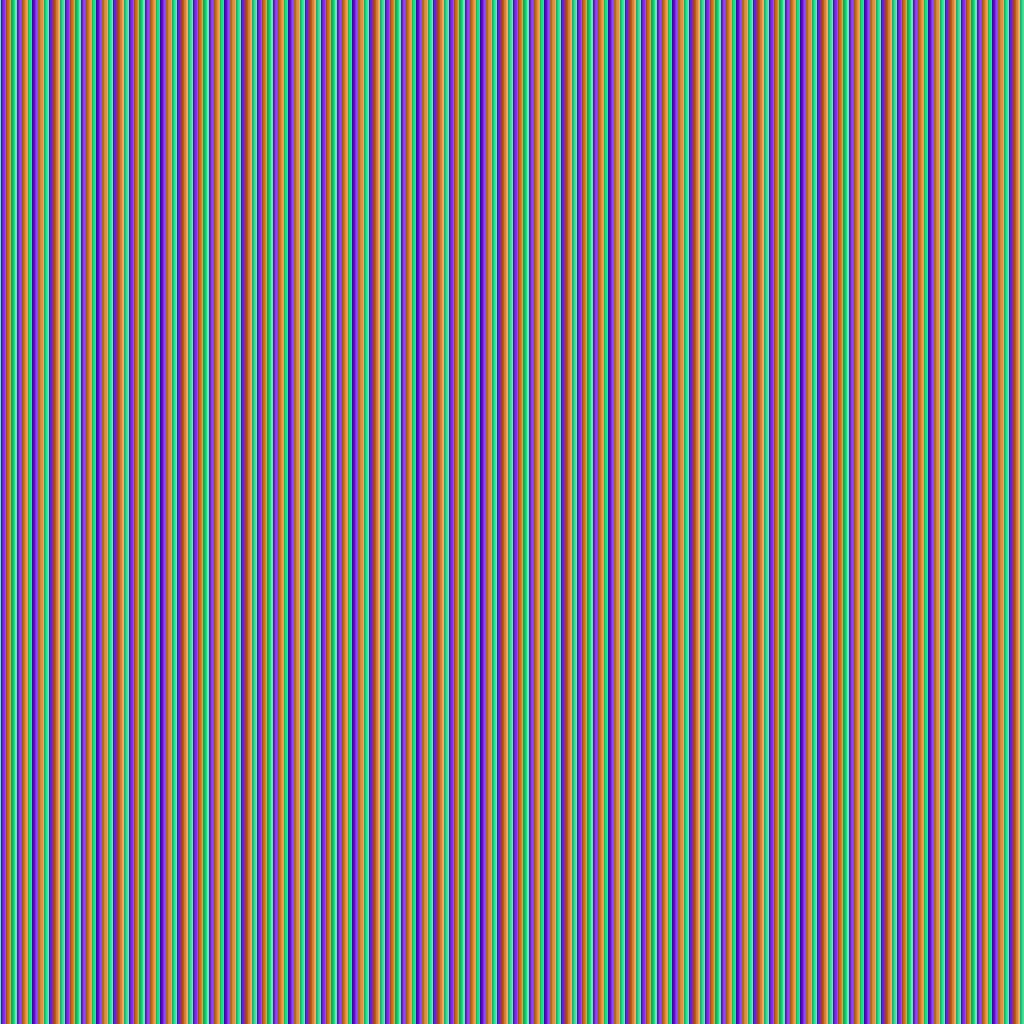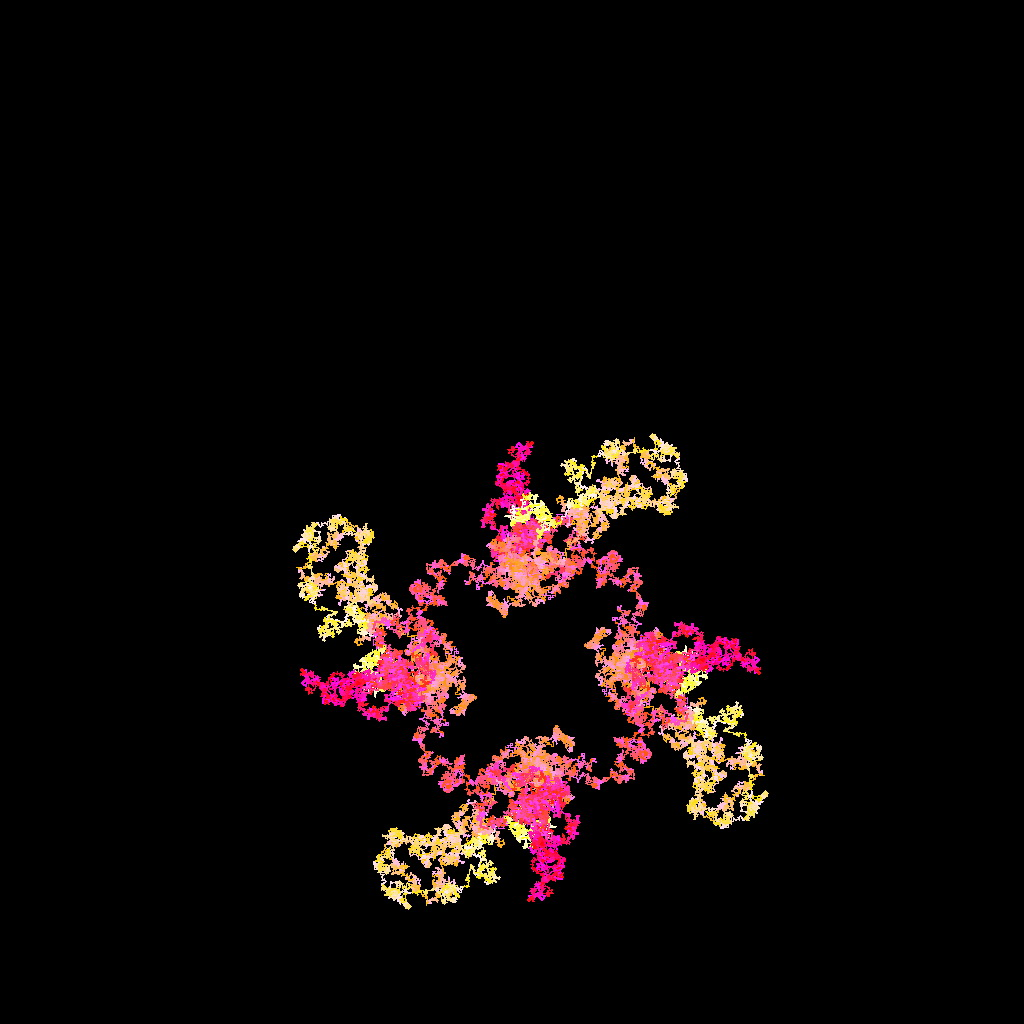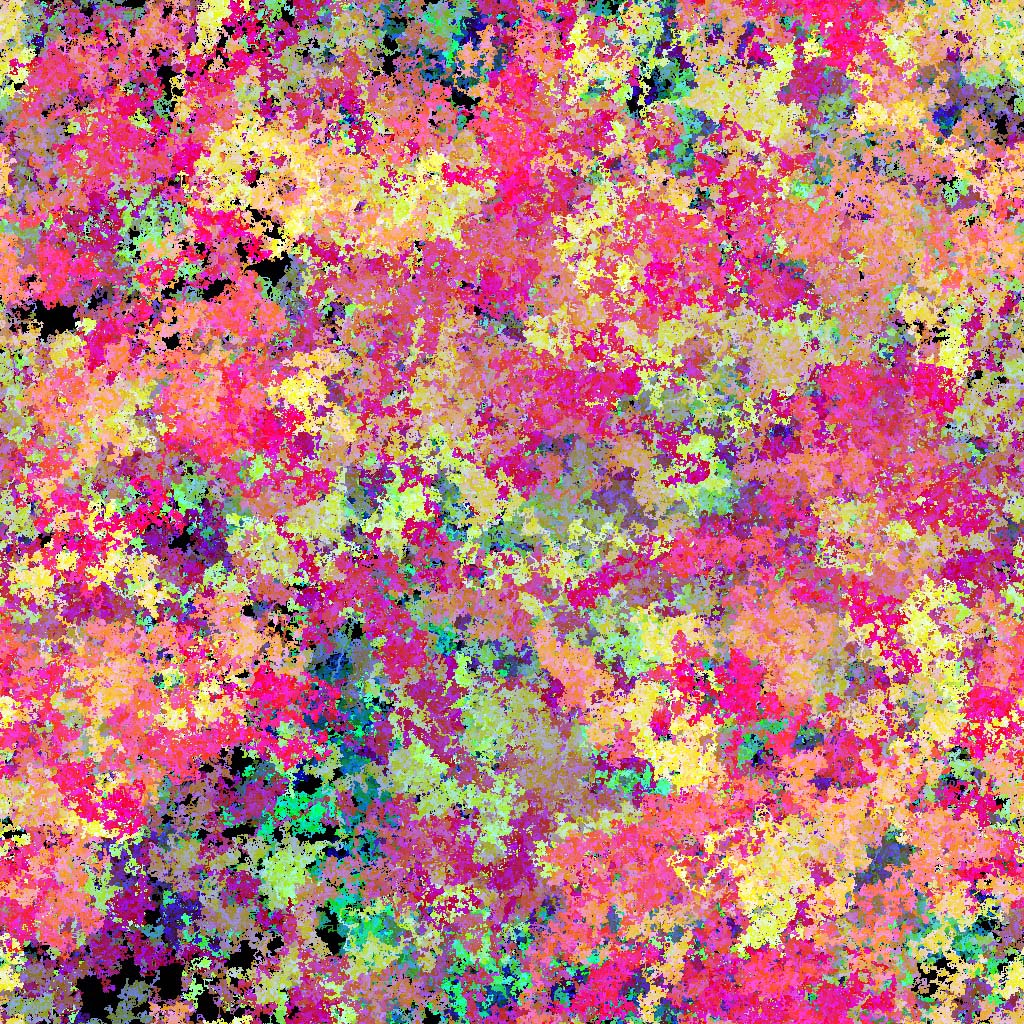# 从图片看出伪随机数的周期性#include<stdio.h>
#include<stdlib.h>
#include<time.h>

int main(){
unsigned int i,s,j;
srand(time(0));
for(i=0;i<100;i++){
s=0;
for(j=0;j<32768;j++){
s+=rand();
}
printf("%d",s%2);
}
return 0;
}


01010101010101010101010101010101……

#include <stdio.h>
#include <stdlib.h>
#include <time.h>
#define DIM 1024

int r[DIM][DIM],g[DIM][DIM],b[DIM][DIM];
FILE *fp;

void init(){
int R,G,B;
int x=DIM/2,y=DIM/2;
srand(time(0));
for(R=0;R<256;R++)for(G=0;G<256;G++)for(B=0;B<256;B++){
r[x][y]=R;g[x][y]=G;b[x][y]=B;
switch(rand()&3){
case 0:y--;break;//up
case 1:x++;break;//right
case 2:y++;break;//down
case 3:x--;break;//left
}
x&=1023;
y&=1023;
}
}

void pixel_write(int i, int j){
static unsigned char color;
color = r[i][j]&255;
color = g[i][j]&255;
color = b[i][j]&255;
fwrite(color, 1, 3, fp);
}

int main(){
init();
fp = fopen("Pic.ppm","wb");
fprintf(fp, "P6\n%d %d\n255\n", DIM, DIM);
for(int j=0;j<DIM;j++)
for(int i=0;i<DIM;i++)
pixel_write(i,j);
fclose(fp);
return 0;
}## 《从图片看出伪随机数的周期性》有7个想法

1. 路人乙说道：

VC++內建的rand()可能是出於效率考量，是使用Linear congruential generator來產生偽隨機數的，GCC改成Mersenne twister了。

如果想在VC++中使用Mersenne twister，要用MT19937

2. Vast Chen说道：

linux下随机数生成比较好。建议试试windows下的 CryptGenRandom（而非cryptRandom ）， 有结果给我发个邮件，谢谢

1. 负一的平方根说道：

我试过了，效果和linux下一样，看不出周期。测试的源代码和效果图已经发邮件了

1. vastchen说道：

收到了，多谢啊。你们的研究真富有艺术。

2. universeCopper说道：

师弟把测试windows下的CryptGenRandom和cryptRandom代码和图片也更新放到这篇文章里吧。

3. USTC路人说道：

师弟好赞！

4. Pingback： Si-Yuan's